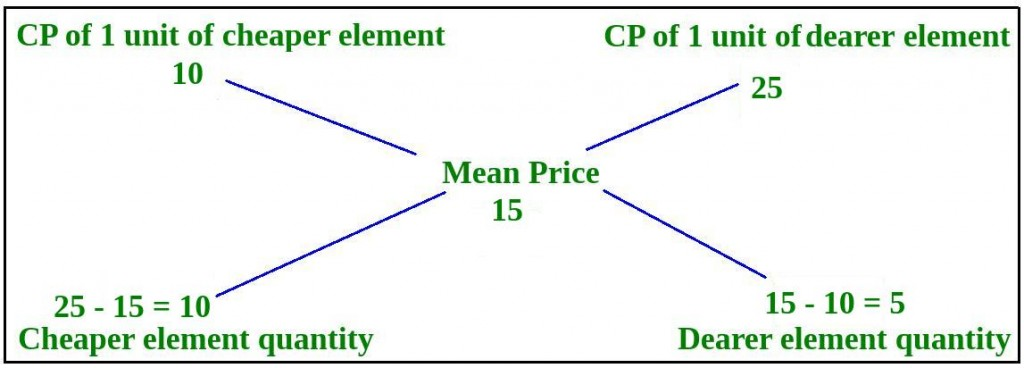# Mixture and Alligation

### Sample Problems

Question 1 : From a vessel of 20 liters pure milk, 1 liter is taken out and replaced with water, so as to keep the volume constant to 20 liters. This process is repeated 5 times. Find the percentage of pure milk left in the vessel after 5 replacements.
Solution : Here, we need to apply the formula P x [1 – (R / P)]n
P = Initial quantity of pure element = 20 liters
R = Quantity replaced every time = 1 liter
n = Number of replacements = 5
So, quantity of pure milk after 5 replacements = 20 x [1 – (1 / 20)]5
Quantity of pure milk after 5 replacements = 20 x (0.95)5 = 20 x 0.773780938 = 15.4756 liters
Therefore, percentage of pure milk left in the vessel after 5 replacements = (15.4756 / 20) x 100 = 77.378 %

Question 2 : A dishonest shopkeeper mixed cheaper quality of rice, priced at Rs. 10 / KG with good quality rice, priced at Rs. 25 / KG and sells the mixture at Rs. 15 / KG. Find the ratio in which he mixes the two qualities of rice.
Solution :Thus, ratio of quantities of cheaper and good quality rice = 10 : 5 = 2 : 1

### Problems on Mixture and Alligation | Set 2

Programs on Mixture and Alligation

Please write comments if you have any doubts related to the topic discussed above, or if you are facing difficulty in any question or if you would like to discuss a question other than those mentioned above.